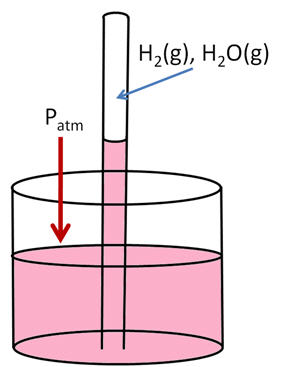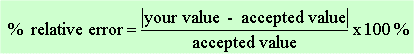### Determination of the Gas Constant, R

Purpose

To derive an experimental value of R and compare it to the published value. A known mass of a metal is reacted with hydrochloric acid to form hydrogen gas, H2. The volume, pressure, temperature and number of moles of the gas are calculated, allowing for the calculation of R.

Introduction

The ideal gas law, PV=nRT can be used to calculate the relative measure quantities of a sample of gas. Knowing three of the four measured quantities (n,P,V and T) we can calculate the fourth if R is known. In this lab we will experimentally determine R by generating a sample of gas and measuring the four quantities and calculating the gas constant by two methods. The procedure for this experiment is based on the chemical reaction between Mg and HCl to produce H2(g):

1 Mg(s) + 2 HCl(aq) ---> 1 MgCl2(aq) +1 H2(g)

The exact mass of Mg will be measured and using the above equation, the moles of H2 produced can be determined. (The reaction goes to completion) The experiment is done in a gas collection tube which is calibrated, so the volume will be easily read. Before measurements are taken, the gas system will be left to equilibrate to the ambient room temperature which can thus be easily measured. Determining the pressure however is a little more challenging. The hydrogen is collected in a eudiometer tube over an aqueous solution and thus after the reaction is complete, the pressure of the gas is that of the produced hydrogen and the vapor pressure of water (which is easy to find in a table somewhere)The liquid levels are the same at the suface of the beaker water, so the pressures on each side are the same. Atmosperic P on one side, H2(g), H2O(g) and a column of water on the other side:  Patm = PH2(g) +  PH2O(g)  + PH2O(l)

You know the pressure of the atmosphere, you look up vapor pressure of water, and you measure the difference in P between the inside of the tube and the atmosphere, as measured by a ruler (PH2O(l) ). Unfortunately, you measured P in mm of H2O, not mm Mg.  To convert the pressure difference in terms of mm of Hg, you must divide mm H2O by 13.546 since Hg is 13.546 times as dense as water at room temperature.

Solve of  PH2(g) and this is called the ‘dry’ pressure of H2(g) since there is no H2O(g) included in the pressure value.  Make sure when you use this equation that all the units are the same (mm Hg).

PV=nRT is called the ideal gas law as it assumes some fundamental properties of gases, namely that the gas molecules have no volume and that their attractive forces are zero which is not always a valid assumption. You will be calculating the value of R using PV=nRT. You will also need to calculate the relative error obtained in your experiment:This report is NOT a short form memo per se. In your report, you need to include all data and calculations in a straight forward and easy to understand fashion. A nice data table with all measured data and intermediately calculated numbers is neede. If you just have numbers (raw data and calculations) you will do poorly. You need to have words to support the data. Once sample calculation of EVERY single calculation you do is required. Think of this as a results section from a short form memo with the raw data included inside. Most of the points are coming from this one section, so be complete! You are calculating a value of R using the ideal gas law for each run. You must report your average value of R, standard devaition as well as percent error. You give only ONE percent error, that from your AVERAGE R value. Also give a short form memo style commentary section.

Prelab questions: (To be written on separately on a piece of recycled paper)

1. What is the vapor pressure of water at 25 deg C ? Where did you get this information?

2. Calculate the value of R using the ideal gas law for the following data: Vdry H2 = 73.52 mL ; Pdry H2 = 635 torr ; massMg (which gets you moles of H2) = 0.0811 g ; T = 23.5 °C.

3. Your instructor will show you how to do setup the experiment, but you must know what information you need to determine for your three runs. Come up with some semblance of a data sheet. You will show this to your instructor on the way in. No data sheet, no lab.

~MEO 23Oct 2006